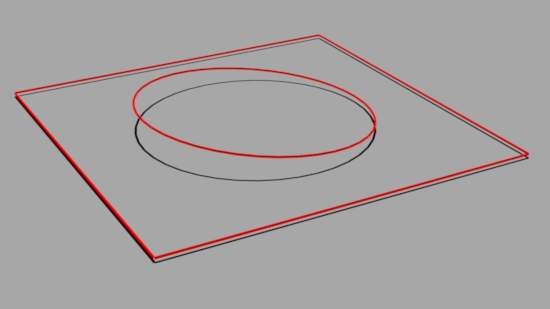### OSLWyvill Lines

return to main index

`Related Tutorial:`
RfM: RiMel - WyvillEdges

#### Introduction

This tutorial presents a shader called `WyvillLines` that uses techniques presented in the following tutorials.
Vectors: Shortest Distance from a Point to a Line
QuadTree: Blobby Geometry
When used to control presence and displacement the shader can produce some interesting results.Figure 1 With and without `WyvillLines`

The shader reads two custom rib attributes that refer to a list of 3d coordinates. For example,

```    Attribute "user" "int verts_count" 
Attribute "user" "point verts_data" [
0.000 1.200 0.000
0.875 2.160 -0.045
0.000 1.200 0.000
-0.537 0.074 0.367
0 0 0
0 0 0
396 additional "padding" xyz coordinates
]```

The `verts_data` attribute specifies a fixed number of 3d coordinates, some which are ignored by the shader. The number of valid coordinates is specified by the `verts_count` attribute. The valid coordinates specify the positions of lines that will control of the effect of the shader. The non-valid coordinates, 0 0 0, merely pad the list so that `verts_data` has a fixed number of coordinates.

##### A Fixed List of Coordinates - Why?

When reading an array of values from an attribute, for example,

```    point data[MAX_VERTICES];
int result = getattribute("user:verts_data", data);```

the size of the array (`data`) that will receive the values must be the same size as the array of values being read by the `getattribute`() function. Hence the need for a fixed array - `MAX_VERTICES` arbitrarily set to 400 in the hope that this will be large enough to handle reasonably complex scenes. However, this means the `verts_data` attribute must, likewise, have 400 coordinates even though in many cases only a relatively small number of coordinates will refer to 3d lines to be used by the shader.

##### How the Coordinates are Used

At each shading point, `WyvillLines` calculates the distance to each line specified by the valid coordinates of `verts_data` and assigns values to it's outputs `resultMask` and `resultF`. If the distance to a line is less than it's `mask_radius` input parameter it assigns 0.0 to `resultMask`. This output is intended to be connected to the "presence" input of PxrSurface.

For example, figure 2 demonstracts effect of using the egdes of a square and a slightly inclined circle to apply "cut" holes in a polygon.Figure 2 With and without holes

 Additioally, the distance to each line is converted to a Wyvill "field function" value. The sum of the field values are assigned to the `resultMask` output. This output is intended to be connected to the scaler input of PxrDisplace. For example, figure 3 demonstracts effect of using the egdes of a square and a slightly inclined circle to apply a displacement to a polygon.Figure 3 With and without displacement

Listing 1 - WyvillLines.osl

 ```// Given a distance squared (dist2), say the distance to a line, and // the radius of influence squared (rad2) the function returns a Wyvill // field value in the range 0 to 1. // See: http://www.fundza.com/algorithmic/quadtree_blobby/index.html float fieldValue(float dist2, float rad2) { if(dist2 >= rad2) return 0.0; float d4 = dist2 * dist2; float d6 = dist2 * d4; float r4 = rad2 * rad2; float r6 = rad2 * r4; return (-0.44444 * d6/r6 + 1.88889 * d4/r4 + -2.44444 * dist2/rad2 + 1); } // Returns the distance from a point and a line. // See: http://fundza.com/vectors/point2line/index.html float distToLine(point p, point start, point end) { vector line_vec = start - end; vector pnt_vec = start - p; float line_len = length(line_vec); vector line_unitvec = normalize(line_vec); vector pnt_vec_scaled = pnt_vec * (1.0/line_len); float delta_t = dot(line_unitvec, pnt_vec_scaled); if(delta_t < 0) delta_t = 0.0; else if(delta_t > 1.0) delta_t = 1.0; point nearest = line_vec * delta_t; return distance(nearest, pnt_vec); } #define MAX_VERTICES 400 // Because arrays in OSL cannot be re-sized the shader declares // a point array of a fixed arbitary length. Although a user attribute // called "verts_data" references MAX_VERTICES of data another user // attribure called "verts_count" specifies the number of valid // vertices. shader WyvillLines(float mask_radius = 0.1, float wyvill_radius = 0.2, int invertMask = 0, output float resultMask = 0, output float resultF = 0) { int len, accumulated_mask = 0; float accumulated_fieldvalue = 0; float wyvill_rad_rad = wyvill_radius * wyvill_radius; point data[MAX_VERTICES]; int result = getattribute("user:verts_count", len); if(result && len <= MAX_VERTICES) { result = getattribute("user:verts_data", data); if(result) { point p = transform("world", P); for(int n = 0; n < len; n += 2) { float dist = distToLine(p, data[n], data[n+1]); if(dist < mask_radius) accumulated_mask++; accumulated_fieldvalue += fieldValue(dist * dist, wyvill_rad_rad); } } } if(invertMask) resultMask = (accumulated_mask > 0) ? 1 : 0; else resultMask = (accumulated_mask > 0) ? 0 : 1; resultF = accumulated_fieldvalue; } ```

© 2002- Malcolm Kesson. All rights reserved.